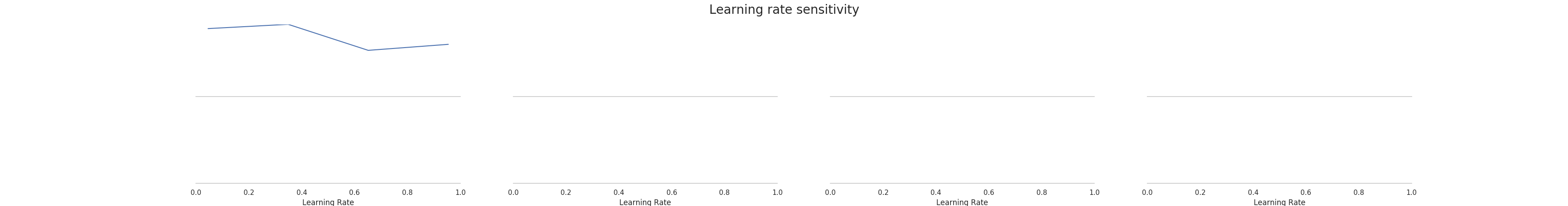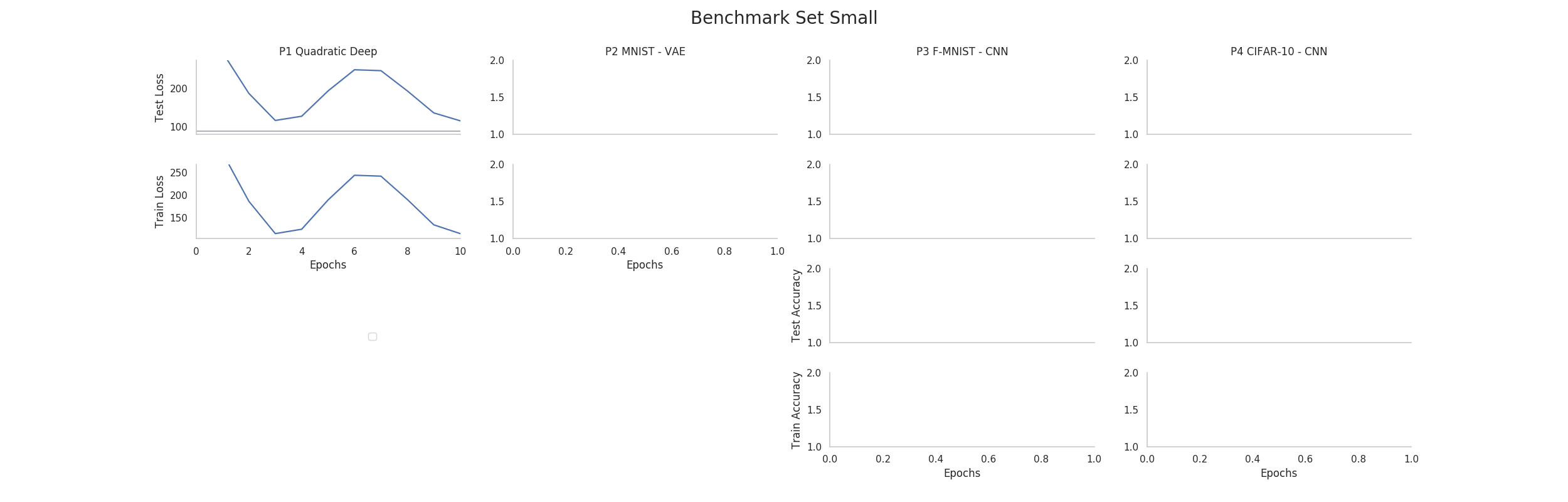# Simple Example¶

This tutorial will show you an example of how DeepOBS can be used to benchmark the performance of a new optimization method for deep learning.

This simple example aims to show you some basic functions of DeepOBS, by creating a run script for a new optimizer (we will use the Momentum optimizer as an example here) and running it on a very simple test problem.

## Create new Run Script¶

The easiest way to use DeepOBS with a new optimizer is to write a run script for it. This run script will import the optimizer and list its hyperparameters (other than the learning rate). For the Momentum optimizer this is simply

momentum_runner.py
  1 2 3 4 5 6 7 8 9 10 11 import tensorflow as tf import deepobs.tensorflow as tfobs optimizer_class = tf.train.MomentumOptimizer hyperparams = [{"name": "momentum", "type": float}, {"name": "use_nesterov", "type": bool, "default": False }] runner = tfobs.runners.StandardRunner(optimizer_class, hyperparams) # The run method accepts all the relevant inputs, all arguments that are not # provided will automatically be grabbed from the command line. runner.run(train_log_interval=10) 

You can download this example run script and use it as a template.

The DeepOBS runner (Line 7) needs access to an optimizer class with the same API as the TensorFlow optimizers and a list of additional hyperparameters for this new optimizers.

This run script is now fully command line based and is able to access all the test problems (and other options) of DeepOBS while also allowing to specify the new optimizers hyperparameters.

## Run new Optimizer¶

Assuming the run script (from the previous section) is called momentum_runner.py we can use it to run the Momentum optimizer on one of the test problems on DeepOBS:

python momentum_runner.py quadratic_deep --bs 128 --lr 1e-2 --momentum 0.99 --num_epochs 10


We will run it a couple times more this time with different learning_rates

python momentum_runner.py quadratic_deep --bs 128 --lr 1e-3 --momentum 0.99 --num_epochs 10
python momentum_runner.py quadratic_deep --bs 128 --lr 1e-4 --momentum 0.99 --num_epochs 10
python momentum_runner.py quadratic_deep --bs 128 --lr 1e-5 --momentum 0.99 --num_epochs 10


## Get best Run¶

We can use DeepOBS to automatically find the best of the hyperparameter settings.

In this example we will directly access the (lower-level) functions of DeepOBS. In the section Suggested Protocol we show you how to use the convenience scripts to do the following steps automatically.

import deepobs

analyzer = deepobs.analyzer.analyze_utils.Analyzer("results")
deepobs.analyzer.analyze.get_best_run(analyzer)


Since all of our results from the previous section are stored in the results folder, we pass this path to the DeepOBS Analyzer. Next, we can call the get_best_run function with this analyzer and get an output like this

***********************
***********************
Checked 4 settings for MomentumOptimizer and found the following
Best Setting (Final Value) num_epochs__10__batch_size__10__momentum__9.9e-01__use_nesterov__False__lr__1.e-04 with final performance of 115.23509434291294
Best Setting (Best Value) num_epochs__10__batch_size__10__momentum__9.9e-01__use_nesterov__False__lr__1.e-03 with best performance of 111.36394282749721


## Plot Results¶

Similarly, we can plot the sensitivity of the (final) performance with regard to the learning rate by calling the appropriate DeepOBS function

deepobs.analyzer.analyze.plot_lr_sensitivity(analyzer)


and getting a plot like thisAnd most importantly, a performance plot of the best performing hyperparameter setting (when looking at the final performance)

deepobs.analyzer.analyze.plot_performance(analyzer, mode='final')